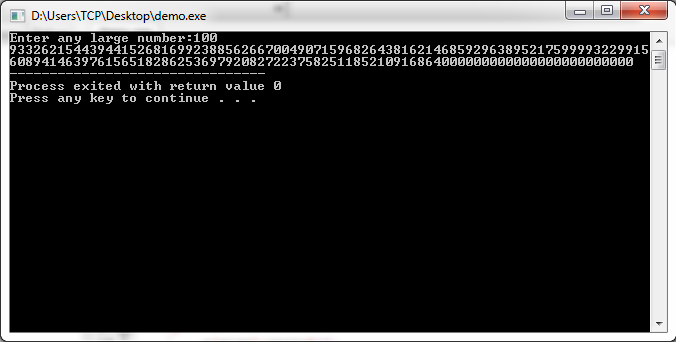# Factorial of Large Number in C and C++

Here you will get program to find factorial of large number in C and C++.

Factorial of big numbers contain so many digits. For example factorial of 100 has almost 158 digits. So there is no data type available to store such a long value. But we can find factorial for large numbers using simple multiplication method that we used in our school time. Below I have shared the program for it.

## Program for Factorial of Large Number in C++

OutputIf you have any doubt regarding above program then you can ask it by commenting below.

### 7 thoughts on “Factorial of Large Number in C and C++”

1.Nice Job.;-) 😉

2.….you have to enlarge array a[ .. ] from 1000 to say 10000 elements – then it’s calculating corectly.

1.yes it will not work for very big numbers like 500!, for that you have to increase the size of array a[]. It is working fine for 100!.

1.Just take a std::vector and enlarge it dynamically. 🙂

3.Nathaniel Cleland

This seems to be a rather space inefficient implementation of bigint. Better would be to store an array of bits and work in base 2, which would be optimised by storing an array of 32 bit integers, storing the result of multiplication as a 64 bit integer to avoid data loss, and adding the overflow to the next column.

4.This is a very nice and popular algorithm to calculate factorial of big number.
But there is a bug with your usage of memory (like other examples in other sites);
There is a waste of memory using an array like a if I want to calculate “small” number,
but even if I digit too big numbers, the array will be insufficient and program will crash.
One solution is use something like this:

int *a;
float stirling = sqrt(2*pi*n)*((n/e)^n);
approx = (int)stirling;
a = malloc(approx * sizeof(int));

where the variable “stirling” contain an approximated size of result stored into the array a[].

5.